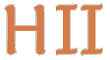# Hilbert II

 English Deutsch
Prototype / Propositional Calculus

The mathematical argumentation is based on logical laws which are developed in the following. The proceeding is thereby strictly formal: it is just manipulating strings by simple rules. The design of the definitions, theorems, propositions, rules and proofs is due to Bernays, Hilbert and Ackermann.

The following documents were generated with the prototype out of qedeq modules. The qedeq modules were checked for formal correctness with the integrated proof verifier. (Also see last column.)

If the viewing of the HTML pages in the first column is not satisfactorily please try the second HTML links (o.html) which require an installed symbol font. If you have also trouble viewing these HTML pages please take look at Ian Hutchinson's pages: Browser Problems and Comparative Review of World-Wide-Web Mathematics Renderers.

#### Basic definitions of propositional and predicate calculus

 lang&rules html o.html informal definition of the used language and the rules of derivation propaxiom html o.html pdf tex qedeq axioms, definitions and declarations of the rules of derivation of propositional calculus

#### Simple derivations

 proptheo1 html o.html pdf tex qedeq first theorems proptheo2 html o.html pdf tex qedeq the axioms of the Whitehead-Russell calculus derived as theorems

#### Systematic development of propositional calculus

Now the elementary theorems of propositional calculus are derived. The basis and master for this is the document deduction. In this document meta rules are already used. Here we use only basis rules therefore the following files are rather big. For shorter and more readable proofs that use meta rules look at the sections below.

 prophilbert1 html o.html pdf tex qedeq first steps prophilbert2 html o.html pdf tex qedeq next level prophilbert3 html o.html pdf tex qedeq third level

#### First meta rule

 subst html o.html pdf tex qedeq declaration of new substitution meta rule

#### Systematic development with meta rules

Same development as before but now meta rules are used. This proceeding is common mathematical methodology and is more close to the document deduction. The above qedeq modules, which don't use meta rules, where automatically generated from the following qedeq modules.

 prophilbert1' html o.html pdf tex qedeq first steps, here further meta rules are declared prophilbert2' html o.html pdf tex qedeq next level prophilbert3' html o.html pdf tex qedeq third level

update 2014-01-20 09:20:48+0100